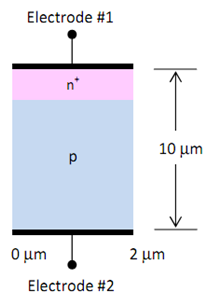## Simulation of a pn junction, Electrical Engineering

Assignment Help:

Simulation of a pn Junction

An n+ p junction is fabricated on a p-type silicon substrate with NA = 8×1015 cm-3 . The n+ region has a concentration of ND = 1.5×1018 cm-3 and a junction depth of xj = 1.4 μm. The total device depth is 10 μm (from top to bottom contact).

Use Sentaurus to simulate and view the results for the following conditions:

1. Zero Bias, Uniform Doping Profiles Using uniform doping profiles, verifies the 1D and 2D doping concentration of this junction. Also plot the 1D potential across the junction to estimate the built-in potential and depletion region width. Compare the results with the theoretical values.

You may want to use a non-uniform mesh, which is denser in the top 2 μm of the device.

2. Reverse Bias, Uniform Doping Profiles Simulate the diode under reverse bias. Plot the reverse IV characteristics and extract the breakdown voltage. Compare your result with Figure in the text. What is the maximum electric field in the junction just before breakdown?3. Forward Bias, Uniform Doping Profiles Plot the IV relation for this diode under a forward bias between 0 and 1 V. What allows us to raise the forward bias above 0.7 V? How can this be seen from the simulation output?

Hint: Examine the change in the IV curve with increasing voltage and determine the cause of this change.

4. High Temperature, Uniform Doping Profiles The junction temperature is raised to 580°C. Simulate this junction up to a reverse bias of 12V and a forward bias of 1V. Plot and explain the IV characteristics.

5. Reverse Bias, Gaussian n+ Doping Profiles Using a Gaussian profile for the n+ region with peak concentration of 1.5×1018 cm-3 and junction depth of xj = 0.7 μm, verify the 1D and 2D doping concentration of the junction. Plot the 1D potential across the junction to estimate the built-in voltage and depletion width at equilibrium. Compare Vbi with the theoretical value and compare Vbi and junction width to those from part 2. Also, estimate the minority carrier diffusion lengths Ln and Lp.

#### Why 8085 processor is called an 8 bit processor, 8085 processor has 8 bit A...

8085 processor has 8 bit ALU (Arithmetic Logic Review). Likewise 8086 processor has 16 bit ALU.

#### Coupling, different coupling methods

different coupling methods

#### Design a circuit using op-amp amplifier, Design a circuit using op-amp that...

Design a circuit using op-amp that will reject the 60kHz power line noise and also reject high signal frequency above 800Khz. The stop-band width around the 60kHz centre frequency

#### Compute the capacitance, Figure shows the cross-section of an electrostatic...

Figure shows the cross-section of an electrostatic problem with translational symmetry: a rectangular coaxial cable. The inner conductor is held at 10 volts and the outer conductor

#### Explain the theory of thermal runaway, Q. Explain the theory of thermal run...

Q. Explain the theory of thermal runaway?           The maximum average power Pdmax which a transistor can dissipate depends upon the transistor construction and may lie in the

.

#### History of transistor, History of Transistor: The great Physicist Juli...

History of Transistor: The great Physicist Julius Edgar Lilienfeld filed the first patent for a transistor in Canada in the year 1925, explaining a device identical to a Field

#### What are the different types of mosfet transistors, Q. What are the differe...

Q. What are the different types of MOSFET transistors ? The metal-oxide semiconductor field-effect transistor (MOSFET) is a three-terminal active device which has many applicat

#### Explain iron and silicon iron alloys, Explain iron and silicon iron alloys....

Explain iron and silicon iron alloys. Iron: Galvanised steel and iron wires that are usually used for earth conductor in low voltage distribution systems may also be utilize

#### Show that the output will be high, Considering the TTL NAND gate circuit of...

Considering the TTL NAND gate circuit of Figure, with one or more inputs low, show that the output will be high.